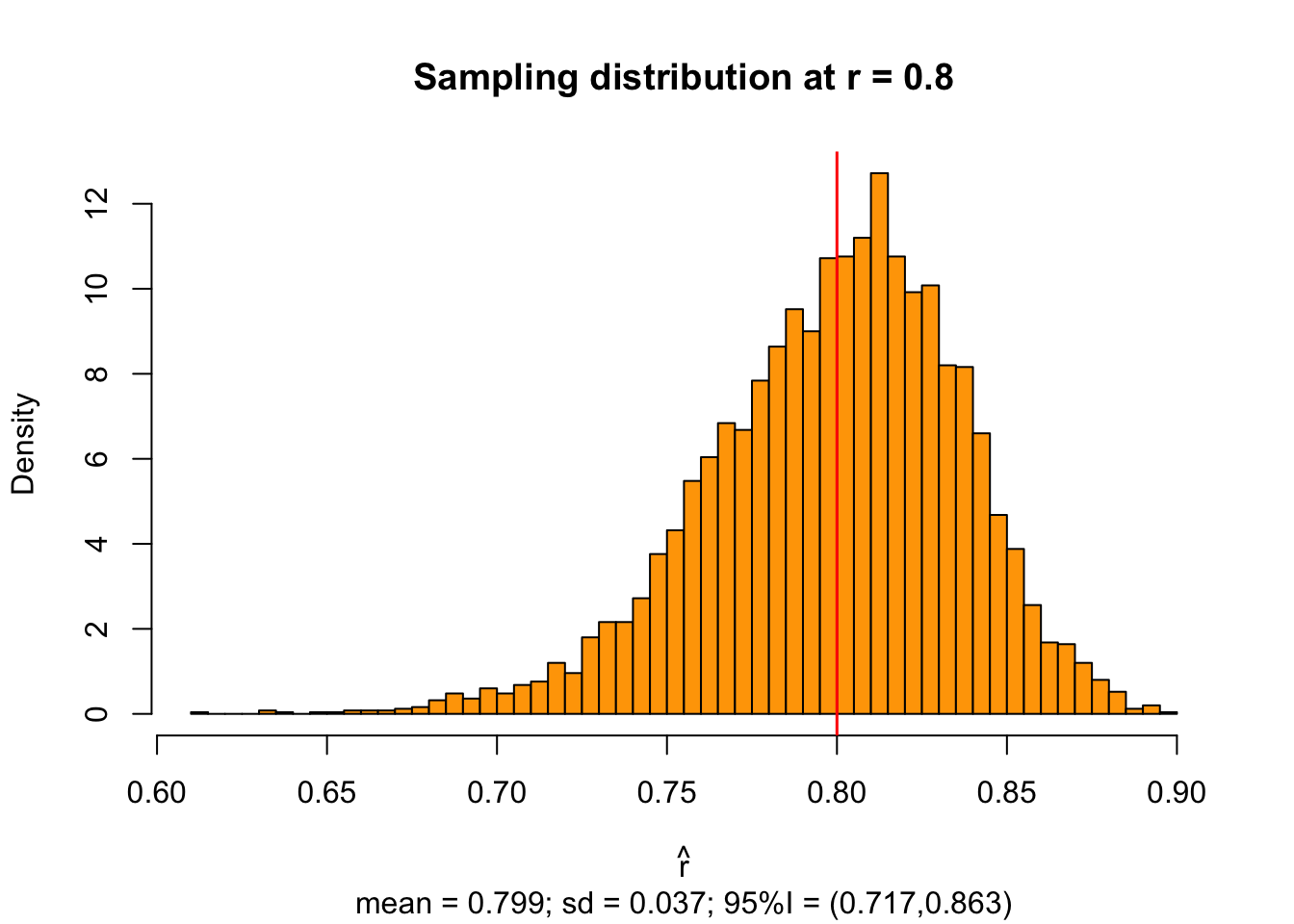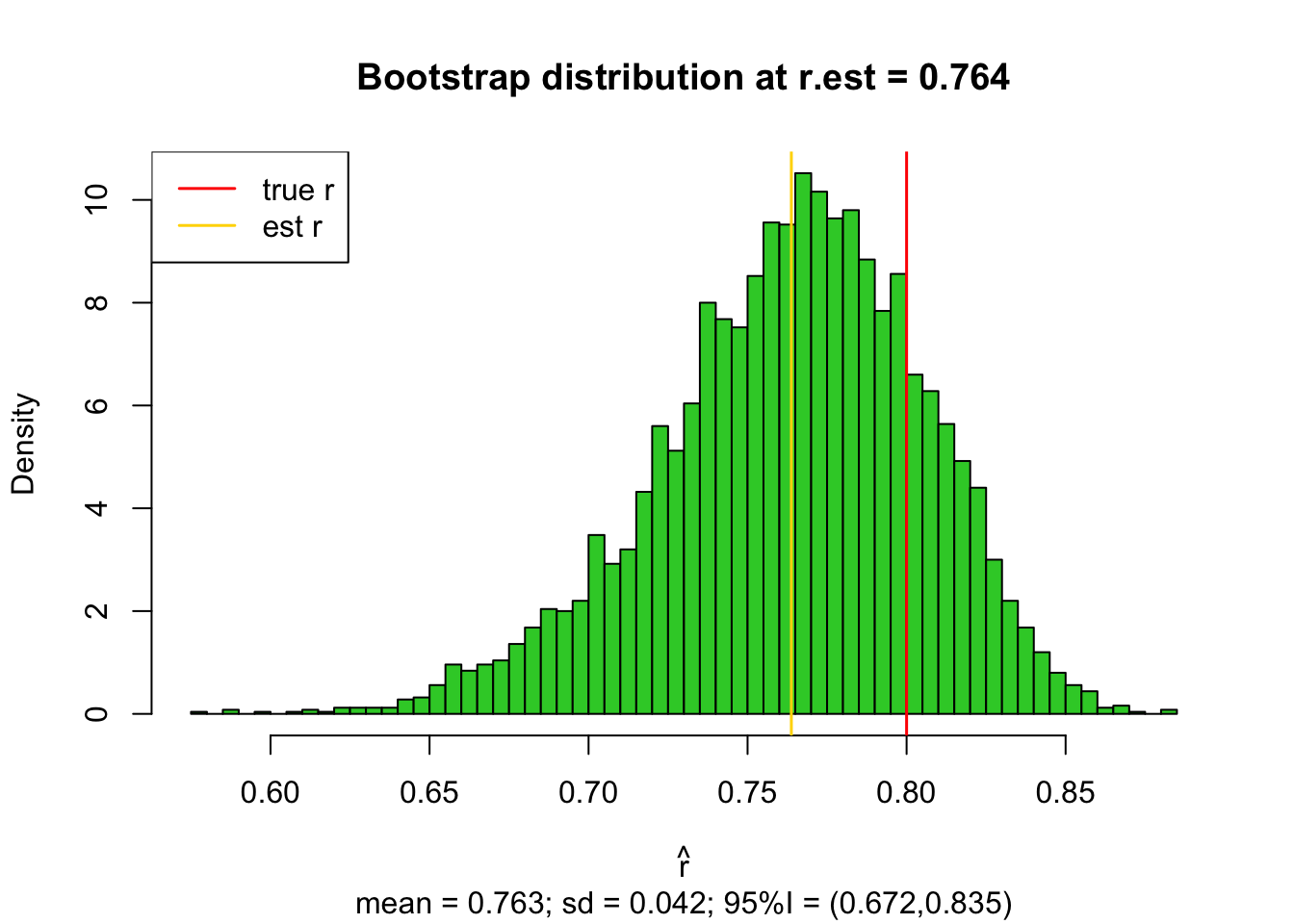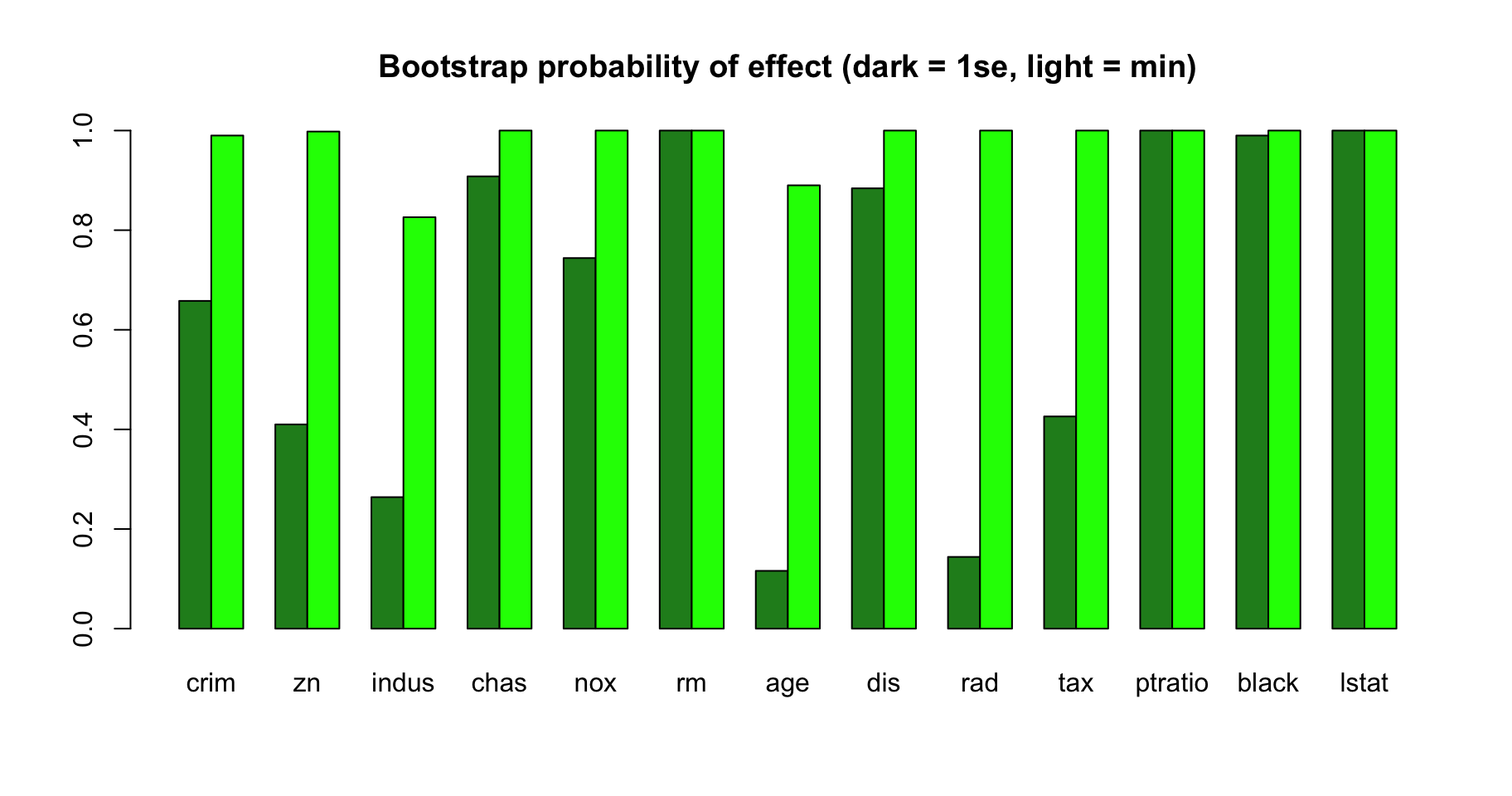From prediction to inference

During the last two weeks, we have been using the predictive ability as the criterion to choose between the regression models. In practice, we have done this via cross-validation that puts the models in an empirical test against data that has not been included in the training data set. This sounds a very reasonable approach to choose models when the goal is to predict well unobserved outcomes.

More broadly, we also intuitively expect that models that are able to best predict the outcome value from the input predictors, are also best in “capturing” the phenomenon we want to study. Therefore, we would also like to extract more information about the phenomenon from those best models than what the point estimates of the coefficients alone give us. A single set of point estimates does not, for example, answer questions such as

• What is our level of confidence that this particular coefficient $$\beta_j$$ is non-zero (probability of being important), or that its value is within a particular interval $$[a,b]$$?
• Are there dependencies between multiple predictors so that they seem to be all needed together for the model to work well? And what are such dependencies in numerical terms?
• Could predictor $$j$$ in the chosen model be equally well replaced by another predictor $$k$$, or some combination of several other predictors?

Answers to such questions require that we quantify our certainty/uncertainty about the chosen model and about the values of the coefficients within the chosen model. In other words, we want to do statistical inference in addition to doing predictions. While there are standard approaches to inference in any one (unpenalized) regression model, such as using the variance-covariance matrix of the ML-estimator to derive confidence intervals/regions, no equally general approach is (yet) available for the penalized models. Here we will approach the problem from two angles:

1. Empirical quantification of variability of our estimation method by using data resampling also known as the bootstrap.
2. Estimating the posterior distribution for the model and its parameters.

The first one, the bootstrap, is a very generally applicable idea of using the observed data set to generate additional possible data sets and quantifying how much variation there is in the parameter estimates of the method when applied across the generated data sets. The flavor of bootstrapping is very practical and it can be interpreted both as an approximate method to estimate the sampling distribution of the parameters of interest and as a Bayesian method to estimate the posterior distribution under certain naive prior distribution about the observed data.

The second approach, the full posterior distribution of all the unknown quantities, is a theoretically sound way to quantify uncertainty, but it is often technically complicated and hence available in special cases rather than in general. A current work on probabilistic programming (e.g. Stan) is aiming to bridge this gap and make the posterior inference more easily available for wider audience.

Bootstrap

Let’s consider the problem of estimating the correlation coefficient $$r$$ between variables $$x$$ and $$y$$ using a sample of size $$n=100$$. We simulate an example where true $$r=0.8$$.

Let’s start by visualizing the sampling distribution of the estimator cor() when it is applied to ($$R=5000$$) independent sets of $$n=100$$ observations from the population. This distribution shows what kind of estimates we are likely to observe in this setting.

#function to generate a pair of correlated variables from lecture 6
gen.cor.pair <- function(r, n){ #r is target correlation, n is sample size
u = rnorm(n, 0, sqrt(abs(r))) #temporary variable that is used for generating x1 and x2
x1 = scale(u + rnorm(n, 0, sqrt(1 - abs(r))))
x2 = scale(sign(r)*u + rnorm(n, 0, sqrt(1 - abs(r))))
cbind(x1, x2) #return matrix
}

n = 100
r.true = 0.8
R = 5000 #replicates of data set
r.est = replicate(R, cor(gen.cor.pair(r = r.true, n = n))[1,2]) #returns cor(x,y) for R indep. data sets
hist(r.est, breaks = 50, main = paste0("Sampling distribution at r = ",r.true), col = "orange",
xlab = expression(hat(r)), prob = T, sub = paste0(
"mean = ",round(mean(r.est),3),"; sd = ",round(sd(r.est),3),"; 95%I = (",
round(quantile(r.est,c(0.025)),3),",",
round(quantile(r.est,c(0.975)),3),")"))
abline(v = r.true, col = "red", lwd = 1.5)We see that with $$n=100$$ the estimates vary from 0.60 to 0.90, with mean close to the true value (in red) but some skew to left.

In real life we do not have a luxury of 5000 independent data sets from which to compute a mean and an interval, but instead we have only one data set of $$n$$ observations. Let’s generate such a single data set.

X = gen.cor.pair(r = r.true, n = n)
r.est = cor(X)[1,2]
r.est
##  0.7637661

This particular data set gives a point estimate of $$\widehat{r}=0.764$$. By observing this value alone we have no idea how much the estimate could vary if we had had additional samples from the population.

If we happen to be aware of inference about correlation coefficient, we can estimate the 95% CI using Fisher’s transformation as

tanh(atanh(r.est)+c(-1,1)*1.96*1/sqrt(n-3)) #95%CI for cor from Fisher's transf.
##  0.6674799 0.8349312

However, Fisher’s transformation is a specific trick applicable only to correlation coefficient estimation and next we will use a more general approach of bootstrap estimation to derive the confidence interval. This happens as follows

1. Resample $$B$$ data sets of size $$n$$, each with replacement from among the original data set $$X$$. These $$B$$ resampled data sets are called bootstrap samples, and denoted by $$X^*_1,\ldots,X_B^*$$ to distinguish them from the original data set $$X$$.

2. Use the distribution of the statistic of interest over the bootstrap samples as a (posterior) distribution for the statistic of interest.

Let’s first see how this works in our case, and then discuss more about the motivation and assumptions behind the procedure.

B = 5000 #bootstrap samples
r.boot = replicate(B, cor(X[sample(1:n, n, replace = T),])[1,2])
hist(r.boot, breaks = 50, main = paste0("Bootstrap distribution at r.est = ",round(r.est,3)),
col = "limegreen", xlab = expression(hat(r)), prob = T,
sub = paste0(
"mean = ",round(mean(r.boot),3),
"; sd = ",round(sd(r.boot),3),
"; 95%I = (", round(quantile(r.boot,c(0.025)),3),",",round(quantile(r.boot,c(0.975)),3),")"))
abline(v = r.true, col = "red", lwd = 1.5)
abline(v = r.est, col = "gold", lwd = 1.5)
legend("topleft", lwd = 1.5, col = c("red","gold"), leg = c("true r","est r"), lty = c(1,1))By interpreting this distribution as a posterior distribution of $$r$$ we can say that a 95% credible interval is (0.672,0.835). Often exactly the same interval is interpreted as a confidence interval so both Bayesian and frequentist interpretations are used for this bootstrap interval.

(Philosophical) Discussion: Even though both Bayesian and frequentist interpretations are used for this interval, there seems not to be good arguments why this interval is a suitable confidence interval, other than acknowledging that it is actually a credible interval that has a good frequentist calibration. For example, a nice treatment of the bootstrap by Hesterberg (2014) p.54 says that “The bootstrap percentile interval has no particular derivation - it just works. This is uncomfortable for a mathematically-trained statistician, and unsatisfying for a mathematical statistics course.” This “problem” may exist with the frequentist interpretation, but does not exist with the Bayesian interpretation, because there this percentile interval of the bootstrap sample is the most obvious estimate for the credible interval.

We see that in our example case this interval agrees well with the Fisher’s transformation above (0.667,0.835), but let’s also do some empirical testing to see how this interval is calibrated over possible other data sets that we could have used for making the bootstrap sample. Let’s make 2000 replicates of the whole bootstrap procedure, each replicate coming from its own data set, and compute the proportion of the replicates where the 95% bootstrap interval contains the true value of $$r$$.

B = 1000
R = 2000
ci.boot = matrix(NA, ncol = 2, nrow = R)
for(ii in 1:R){
X = gen.cor.pair(r = r.true, n = n) #generate one pair of predictors
r.boot = replicate(B, cor(X[sample(1:n, n, replace = T),])[1,2])
ci.boot[ii,] = quantile(r.boot,c(0.025,0.975))
}
#Count in which proportion of data sets the bootstrap 95%CI contained the true r
mean(ci.boot[,1] <= r.true & r.true <= ci.boot[,2])
##  0.9335

We have pretty well-calibrated 95% intervals (in frequentist sense) from the bootstrap samples, meaning that ~95% of the intervals indeed contain the true parameter. (To be precise, here the intervals are a bit narrow with coverage of about 93.4% but it still gives a good idea where 95% of the mass is.)

To conclude: Based on the single data set we estimated $$\widehat{r}=0.764$$, (we don’t use the bootstrap sample for this point estimate but only the cor() applied to our original data set), with a 95% credible (or confidence) interval of (0.67,0.84).

What exactly is the bootstrap used for ?

The term “bootstrap” (likely) originates from the saying “to pull oneself up by one’s bootstraps”, which means to complete an impossible task. Bradley Efron introduced the term in his 1979 paper about the method. The “impossible-sounding” task here is to estimate the sampling distribution of the estimator by using only one available estimate. The reason that the task is not quite as impossible as it may sound is that the single available estimate results from a data set of $$n$$ data points, and the variation of the estimator can be approximated by resampling additional data sets of the same size as the original data set ($$n$$), using the available data set as an approximation to the target population.

We use bootsrap to estimate the uncertainty in our statistic of interest. Typical cases are to use SD of the bootstrap sample to estimate SE of the parameter estimate and to use the $$\alpha\cdot 100$$% interval of bootstrap sample to approximate the corresponding credible/confidence interval for the parameter. Bootstrap works better, the more symmetric and unbiased the sampling distribution is, and bootstrap can fail if the shape of the distribution very strongly depends on the parameter value, or if the sample size is very small.

Note that we do not use bootstrap to try to somehow make our single point estimate more accurate. Such a task indeed remains impossible! By resampling from the existing data set we cannot create more accuracy about the parameter value compared to what we already have extracted from the available data set. Bootstrap simply helps us to approximate how much our point estimate might vary if we had observed another data set of similar size from the same population (frequentist interpretation), or to approximate the posterior distribution of the parameter of interest (Bayesian interpretation).

Bootstrap resamples with replacement

When the original data is $$X=(x_1,\ldots,x_n)$$, a bootstrap sample $$X^*=(x_1^*,\ldots,x_n^*)$$ is a sample of size $$n$$ from the original data, where each $$x_j^*$$ has been sampled independently from among the $$n$$ values of $$X$$, with equal probability $$\frac{1}{n}$$ given for each data point $$x_k$$ of $$X$$. In other words, for any bootstrap sample $$X^*$$, $$\textrm{Pr}(x_j^* = x_k) = \frac{1}{n}$$ for all pairs $$j,k\in\{1,\ldots, n\}$$.

In particular, it follows that the bootstrap sample can include a single data point $$x_k$$ many times and can miss some other value $$x_{k'}$$ completely. Actually, the probability that a bootstrap sample misses a particular data point $$x_k$$ is $$(1-\frac{1}{n})^n \underset{n\to\infty}{\longrightarrow}e^{-1}\approx 0.368.$$ Thus, in large samples, only about 63.2% of the data points are included in any one bootstrap sample.

For example, if we have observed data set $$X=(0.6,1.3,2.0)$$ we could make $$3^3=27$$ bootstrap samples, including, e.g., $$(0.6,0.6,0.6)$$,$$(1.3,2.0,1.3)$$ and $$(2.0, 0.6, 1.3)$$.

Why does bootstrap work?

The non-parameteric bootstrap, that we have been considering, can be seen as first building an estimate for the probability distribution $$f(x)$$ of the data $$x$$ in the target population, and second using that estimate of the distribution for inference about the statistic of interest, $$\theta = \theta(x)$$, in the target distribution.

Thus bootstrap does inference based on the assumption that the empirical distribution $$\widehat{f}$$ of the data points observed in the data set $$X$$ is a good approximation to the complete target distribution of the data points that could have been sampled / will be sampled in the future. This empirical distribution simply puts a point mass of $$\frac{1}{n}$$ on each observed data point and assumes that no other data points are possible. It is clear that this is a crude approximation, and depends on the situation and sample size how good results it gives.

A Bayesian interpretation of bootstrap, first framed by Rubin in 1981, assumes that we are estimating the discrete probability of observing each possible data point. We put an uninformative prior distribution on the probabilities of all possible data points and then we update the probabilities as we observe new data points. Under this very naive model, the expected value of the posterior distribution after observing $$n$$ data points is the bootstrap distribution where each observed point has probability of $$\frac{1}{n}$$, and there is 0 probability for any so far unobserved data point. It follows that the posterior distribution for any given statistic $$\theta = \theta(x)$$ can then be approximated by the bootstrap method as described above. A detailed explanation is given by Rasmus Bååth.

Bootstrap with penalized regression

Let’s apply the bootstrap to a regression setting. Let’s predict medv (median price in a region) from the other variables available in Boston data set. We will first standardize all the variables.

The results from LASSO are:

library(MASS) #include Boston data set
library(glmnet)
y = scale(Boston$medv) X = scale(as.matrix(Boston[,-which(names(Boston) == "medv")])) #Let's apply the standard LASSO estimation at lambda.1se cv.lasso = cv.glmnet(x = X, y = y, alpha = 1) lasso.coef = as.numeric(coef(cv.lasso$glmnet.fit, s = cv.lasso$lambda.1se)) cv.lasso$lambda.1se
##  0.02839794
data.frame(lasso.coef, row.names = c("intcept", colnames(X)))
##            lasso.coef
## intcept -2.044123e-16
## crim    -3.028936e-02
## zn       2.129334e-02
## indus    0.000000e+00
## chas     6.199843e-02
## nox     -9.238078e-02
## rm       3.247971e-01
## age      0.000000e+00
## dis     -1.445185e-01
## tax      0.000000e+00
## ptratio -1.919869e-01
## black    7.024556e-02
## lstat   -4.036471e-01

Here four coefficients are set to 0. How confident we are about these being 0? Let’s see whether the same four coefficients are 0 also in all/most/some bootstrap samples. We will simply bootstrap the rows of the Boston data set, fit LASSO model and collect the lambda parameter and all the coefficients. We will do this also for lambda.min simultaneously.

n = length(y)
B = 500 #bootstrap samples
boot.coef.1se = matrix(NA, nrow = B, ncol = ncol(X) + 1) #+1 for the intercept
colnames(boot.coef.1se) = c("intcept", colnames(X))
boot.coef.min = boot.coef.1se
boot.lambda = matrix(NA, nrow = B, ncol = 2)
colnames(boot.lambda) = c("1se","min")
for(ii in 1:B){
boot.ind = sample(1:n, size = n, replace = T) #bootstrap indexes
XX = X[boot.ind,]
yy = y[boot.ind]
boot.cv.lasso = cv.glmnet(x = XX, y = yy, alpha = 1)
boot.coef.1se[ii,] = as.numeric(coef(boot.cv.lasso$glmnet.fit, s = boot.cv.lasso$lambda.1se))
boot.coef.min[ii,] = as.numeric(coef(boot.cv.lasso$glmnet.fit, s = boot.cv.lasso$lambda.min))
boot.lambda[ii,] = c(boot.cv.lasso$lambda.1se, boot.cv.lasso$lambda.min)
}
prob.nonzero.1se = apply(abs(boot.coef.1se) > 1e-6, 2, mean) #proportion where each coef was > 1e-6
prob.nonzero.min = apply(abs(boot.coef.min) > 1e-6, 2, mean)

barplot(rbind(prob.nonzero.1se[-1],prob.nonzero.min[-1]), beside = T,
col = c("forestgreen", "green1"),
main = "Bootstrap probability of effect (dark = 1se, light = min)")Looking at the lambda.1se values (dark green), we see that none of the four predictors are always 0, although they are among the ones having the smallest effect probabilities: age and rad ~10%, indus ~20% and tax and zn ~40%. On the other hand, lstat, ptratio and rm seem to be always non-zero.

At lambda.min we see that only age and indus are sometimes set to near zero (< 1e-6) whereas in most cases all variables are having coefficients of larger magnitude.

How much estimated lambda has varied across bootstrap?

summary(boot.lambda)
##       1se               min
##  Min.   :0.01533   Min.   :0.0005163
##  1st Qu.:0.03072   1st Qu.:0.0007457
##  Median :0.03810   Median :0.0008656
##  Mean   :0.04153   Mean   :0.0013371
##  3rd Qu.:0.04723   3rd Qu.:0.0017203
##  Max.   :0.13161   Max.   :0.0058687

Not very much and we see that lambda.min is often very close to zero meaning that cross-validation suggests that there is little need for shrinkage in these data.

We can now also look at the variation in the values of coefficients.

par(mfrow = c(2, 1))
par(mar = c(1, 3, 1, 1))
boxplot(boot.coef.1se[,-1], col = "forestgreen", main = "",
xaxt = "n", ylim = c(-0.6, 0.4), cex = 0.5)
abline(h = 0, lty = 2)
text(1.7, 0.35, "lambda.1se", cex = 1.4, col = "forestgreen")
par(mar = c(2, 3, 0, 1))
boxplot(boot.coef.min[,-1], col = "green1", main = "", ylim = c(-0.6, 0.4), cex = 0.5)
abline( h = 0, lty = 2)
text(1.7, 0.35, "lambda.min", cex = 1.4, col = "green1")